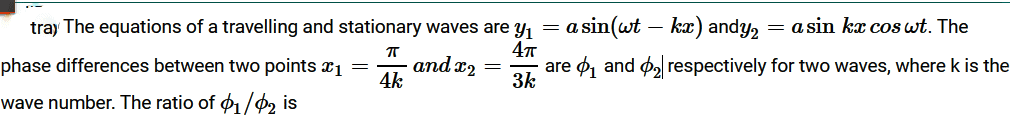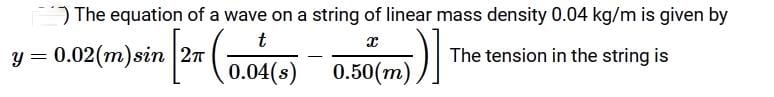JEE  >  QUIZ 2:Waves(#Free Test Series)

# QUIZ 2:Waves(#Free Test Series)

Test Description

## 15 Questions MCQ Test | QUIZ 2:Waves(#Free Test Series)

QUIZ 2:Waves(#Free Test Series) for JEE 2023 is part of JEE preparation. The QUIZ 2:Waves(#Free Test Series) questions and answers have been prepared according to the JEE exam syllabus.The QUIZ 2:Waves(#Free Test Series) MCQs are made for JEE 2023 Exam. Find important definitions, questions, notes, meanings, examples, exercises, MCQs and online tests for QUIZ 2:Waves(#Free Test Series) below.
Solutions of QUIZ 2:Waves(#Free Test Series) questions in English are available as part of our course for JEE & QUIZ 2:Waves(#Free Test Series) solutions in Hindi for JEE course. Download more important topics, notes, lectures and mock test series for JEE Exam by signing up for free. Attempt QUIZ 2:Waves(#Free Test Series) | 15 questions in 20 minutes | Mock test for JEE preparation | Free important questions MCQ to study for JEE Exam | Download free PDF with solutions
 1 Crore+ students have signed up on EduRev. Have you?
QUIZ 2:Waves(#Free Test Series) - Question 1

###QUIZ 2:Waves(#Free Test Series) - Question 2

###QUIZ 2:Waves(#Free Test Series) - Question 3

### A person is standing at a distance D from an isotropic point source of sound. He walks 50.0 m towards the source and observes that the intensity of the sound has doubled. His initial distance D from the source is

Detailed Solution for QUIZ 2:Waves(#Free Test Series) - Question 3

nearly 170.7m

QUIZ 2:Waves(#Free Test Series) - Question 4

How long will it take sound waves to travel a distance between points A and B if the air temperature between them varies linearly from T1 toT2? (The velocity of sound in air at temperature T is given by v=α√t, where a is a constant)

QUIZ 2:Waves(#Free Test Series) - Question 5

Two sources S1 and S2 of same frequencyfemits sound. The sources are moving as shown with speed u each. A stationary observer hears that sound. The beat frequency is (v = velocity of sound)

*Answer can only contain numeric values
QUIZ 2:Waves(#Free Test Series) - Question 6

A wire of density 9×10kg/m3 is stretched between two clamps 1 m apart and is subjected to an extension of 4.9×10-4 m. What is the lowest frequency (in Hz) of transverse vibration in the wire?

*Answer can only contain numeric values
QUIZ 2:Waves(#Free Test Series) - Question 7

The difference between the apparent frequency of a source of sound as perceived by an observer during its approach and recession is 2% of the natural frequency of the source. If the velocity of sound in air is 300 m/sec, what is the velocity (in m/s) of the source? (It is given that velocity of source << velocity of sound)

*Answer can only contain numeric values
QUIZ 2:Waves(#Free Test Series) - Question 8

Two identical sounds S1 and S2 reach at a point P in phase. The resultant loudness at point P is ndB higher than the loudness of S1. What is the value of n?

Detailed Solution for QUIZ 2:Waves(#Free Test Series) - Question 8

*Answer can only contain numeric values
QUIZ 2:Waves(#Free Test Series) - Question 9

Wavelengths of two notes in air are 1 m and1/164m. Each note produces 1 beat/s with a third note of a fixed frequency. What is the speed (in m/s) of sound in air?

*Answer can only contain numeric values
QUIZ 2:Waves(#Free Test Series) - Question 10

The displacementyof a particle executing periodic motions given byy=4cos2t/2(sin1000t).How many independent harmonic motions may be considered to superpose to result this expression.

QUIZ 2:Waves(#Free Test Series) - Question 11

A tube closed at one end and containing air is excited. It produces the fundamental note of frequency 512 Hz. If the same tube is open at both the ends the fundamental frequency that can be produced is [RPET 1999]

Detailed Solution for QUIZ 2:Waves(#Free Test Series) - Question 11
•  Fundamental frequency of open pipe is double that of the closed pipe.

QUIZ 2:Waves(#Free Test Series) - Question 12

A closed pipe and an open pipe have their first overtones identical in frequency. Their lengths are in the ratio [Roorkee 1999]

QUIZ 2:Waves(#Free Test Series) - Question 13

The first overtone in a closed pipe has a frequency [JIPMER 1999]

QUIZ 2:Waves(#Free Test Series) - Question 14

An empty vessel is partially filled with water, then the frequency of vibration of air column in the vessel [KCET 2000]

QUIZ 2:Waves(#Free Test Series) - Question 15

It is desired to increase the fundamental resonance frequency in a tube which is closed at one end. This can be achieved by [Roorkee 2000]

 Use Code STAYHOME200 and get INR 200 additional OFF Use Coupon Code
Information about QUIZ 2:Waves(#Free Test Series) Page
In this test you can find the Exam questions for QUIZ 2:Waves(#Free Test Series) solved & explained in the simplest way possible. Besides giving Questions and answers for QUIZ 2:Waves(#Free Test Series), EduRev gives you an ample number of Online tests for practice